# KSEEB Solutions for Class 9 Maths Chapter 12 Circles Ex 12.5

In this chapter, we provide KSEEB SSLC Class 9 Maths Chapter 12 Circles Ex 12.5 for English medium students, Which will very helpful for every student in their exams. Students can download the latest KSEEB SSLC Class 9 Maths Chapter 12 Circles Ex 12.5 pdf, free KSEEB SSLC Class 9 Maths Chapter 12 Circles Ex 12.5 pdf download. Now you will get step by step solution to each question.

## Karnataka Board Class 9 Maths Chapter 12 Circles Ex 12.5

Question 1.
In Fig., A, B, and C are three points on a circle with centre O such that ∠BOC = 30° and ∠AOB = 60°. If D is a point on the circle other than the arc ABC, find ∠ADC.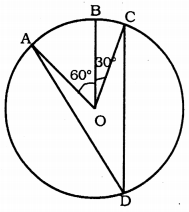Solution:
∠AOB + ∠BOC = 60° + 30° = 90°
∴ ∠AOC = 90° (Angle subtended at the centre)
The angle subtended by an arc at the centre is double the angle subtended by it at any point on the remaining part of the circle.
∴ Angle subtended at the centre
90° = 2 × ∠ADC 90
∴ ∠ADC = 902 = 45°

Question 2.
A chord of a circle is equal to the radius of the circle. Find the angle subtended by the chord at a point on the minor arc and also at a point on the major arc.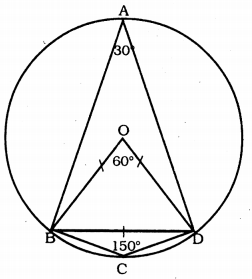Solution:
(i) Angle subtended in the circumference, ∠BAD =?
(ii) Angle subtended in the circumference, ∠BCD =?
In this figure BD is a chord, OB is the radius, it is equal to OD.
∴ OB = OD = BD
∴ ∆OBD is equilateral triangle.
∴ each angle is equal to 60°.
∴ angle subtended at the centre ∠BOD = 60°.
(i) Angle subtended in the circumference
∠BAD= 12 × angle subtended at centre ∠BOD
= 12 × 60°

(ii) The sum of either pair of opposite angles of a cyclic quadrilateral is 180°.
30 + ∠ACD = 180 (∵ ∠BAD = 30°)
∴ ∠ADC = 180 – 30
∴ ∠ACD = 150°.

Question 3.
In Fig., ∠PQR = 100°. where P, Q and R are points on a circle with centre O. Find ∠OPR.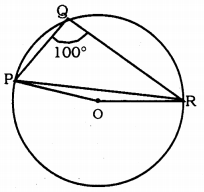Solution:
If ∠PQR = 100°, then ∠OPR = ?
∠PQR = 100°.
∴ ∠POR = ?
∠POR = 2 × ∠PQR = 2 × 100°
∠POR = 200°
∴ ∠POR – Reflex angle ∠POR = 360°
∴ ∠POR = 360 – 200
∴ ∠POR = 160°
In ∆POR, OP = OR radii.
∴ ∠OPR = ∠ORB
∴ ∠OPR + ∠ORP + ∠POR = 180°
∠OPR + ∠OPR + 160 = 180
2∠OPR+ 160 = 180
2∠OPR = 180 – 160
2∠OPR = 20
∠OPR = 202
∴ ∠OPR= 10°.

Question 4.
In Fig., ∠ABC = 69°, ∠ACB = 31°, find ∠BDC.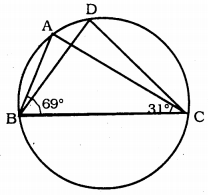Solution:
If ∠ABC = 69°, ∠ACB = 31°, then ∠BDC = ?
In ∆ABC,
∠ABC = ∠ACB + ∠BAC = 180°
69 + 31 + ∠BAC = 180
100 + ∠BAC = 180
∠BAC = 180 – 100
∠BAC = 80°
∠BAC and ∠BDC are angles in same segment. These are equal.
∠BDC = ∠BAC = 80°
∴ ∠BDC = 80°.

Question 5.
In Fig., A, B, C, and D are four points on a circle. AC and BD intersect at a point E such that ∠BEC = 130° and ∠ECD = 20°. Find ∠BAC.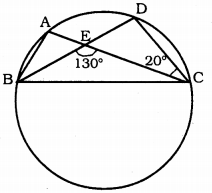Solution:
∠BEC = 130°, ∠ECD = 20°, ∠BAC = ?
Angles formed by arc AD are ∠ABD, ∠ACD is equal.
∴ ∠ABD = ∠ACB = 20°
∠ABD = 20°
∠AEB + ∠BEC = 180 (adjacent angles)
∠AEB + 130° = 180
∠AEB = 180 – 130
∴ ∠AEB = 50°
Now, in ∆BAE,
∠BAE = ∠ABE + ∠AEB = 180°
∠BAE + 20 + 50 = 180
∠BAE + 70 = 180
∠BAE = 180 – 70
∴ ∠BAE = 110°
But ∠BAE and ∠BAC are the same.
∴ ∠BAC = 110°

Question 6.
ABCD is a cyclic quadrilateral whose diagonals intersect at a point E. If ∠DBC = 70°, ∠BAC = 30°, find ∠BCD. Further, if AB = BC, find ∠ECD.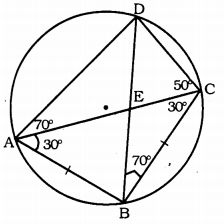Solution:
∠DBC = 70°, ∠BAC = 30°, then ∠BCD =?
AB = BC, then ∠ECD = ?
∠DAC and ∠DBC are angles in the same segment.
∴ ∠DAC = ∠DBC = 70°
∴ ∠DAC = 70°
∴ Sum of opposite angles is 180°.
∠DAB + ∠DCB = 180
100 + ∠DCB = 180
[∵ ∠DAC + ∠BAC = ∠DAB 70 + 30 = 100]
∠DCB = 180 – 100
∴ ∠DCB = 80
∠DCB = ∠BCD = 80
∴ ∠BCD = 80
In ∆ABC, AB = AC,
∴ ∠BAC = ∠BCA = 30°
∠BCA = 30°
∠ECD = ∠BCD – ∠BCA = 80 – 30
∴ ∠ECD = 50°.

Question 7.
If diagonals of a cyclic quadrilateral are diameters of the circle through the vertices of the quadrilateral, prove that it is a rectangle.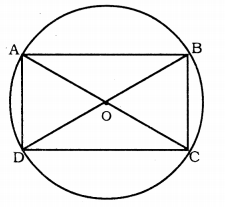Solution:
Data: In cyclic quadrilateral ABCD, AC and BD are diameters of circle.
To Prove: ABCD is a rectangle.
Proof: AC is a diameter. ∠ABC is angle in semicircle. Angle in semicircle is a right angle.
∴ ∠ABC = 90° ∠ADC = 90°
Similarly, BD is a diamgers, ∠DAB, ∠DCB are angles in semicircle.
∠DAB = 90° ∠DCB = 90°
Now, four angles of quadrilateral ABCD are right angles.
∴ ∠A = ∠B = ∠C = ∠D = 90°
∴ ABCD is a rectangle.

Question 8.
If the non-parallel sides of a trapezium are equal, prove that it is cyclic.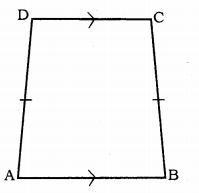Solution:
Data: ABCD is a trapezium, DC || AB and AD = BC which are non-parallel sides.
To Prove: ABCD is a cyclic quadrilateral.
Proof: ABCD is a trapezium.
AB || CD and AD = BC.
∴ ∠DAB + ∠CDA = 180° …………. (i)
(sum of interior angles)
Similarly, ∠DCB + ∠ABC = 180° …………. (ii)
As we know, AD = BC,
∴∠DAB = ∠CBA
Substituting Eqn. (i) in Eqn. (ii),
∠CBA + ∠CDA = 180°
∠DAB + ∠DCB = 180°
If sum of angles of a quadrilateral is 180°, then it is cyclic quadrilateral.
∴ ABCD is a cyclic quadrilateral.

Question 9.
Two circles intersect at two points B and C. Through B, two line segments ABD and PBQ are drawn to intersect the circles at A, D and P, Q respectively. Prove that ∠ACP = ∠QCD.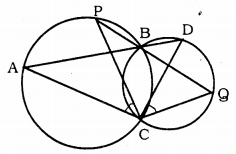Solution:
Two circles are drawn taking PQ and PR of a triangle as diameter. Let these intersect at P and S.
To Prove: ∠ACP = ∠QCD
Proof: ∠ABP = ∠QBD ………….. (i) (vertically opposite angles)
∠ABP = ∠ACP ……….. (ii) (angles in the same segment)
Similarly, ∠QCD = ∠QBD …………. (iii)(angles in the same segment)
From (i), (ii), and (iii),
∠ACP = ∠QCD.

Question 10.
If circles are drawn taking two sides of a triangle as diameters, prove that the point of intersection of these circles lie on the third side.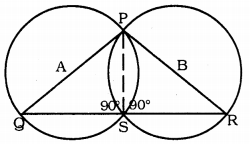Solution:
Data: Two circles are drawn taking PQ and PR of a triangle as diameter. Let these intersect at P and S.
To Prove: The point of intersection ‘S’ is on the third side QR of ∆PQR.
Construction: Join PS.
Proof: QAP is a diameter.
∴ ∠QSP = 90° (angle in the semi-circle) Similarly, ABR is a diameter.
∠PSR – 90° (angle in the semicircle)
∠QSR = ∠QSP + ∠RSP = 90 + 90
∠QSR = 180°
∴ ∠QSR is a straight angle.
∴ QSR is a straight line.
∴ Point ‘S’ is on the third side QR of ∆PQR.

Question 11.
∆ABC and ∆ADC are two right triangles with common hypotenuse AC. Prove that ∠CAD = ∠CBD.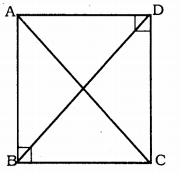Solution:
Data: ∆ABC and ∆ADC are right-angled triangles having common hypotenuse AC.
Proof: In ∆ABC, ∠ABC = 90°
∴ ∠BAC + ∠BCA = 90° …………. (i)
∴ ∠DAC + ∠DCA = 90° …………… (ii)
∠BAC + ∠BCA + ∠DAC + ∠DCA = 90 + 90
(∠BAC + ∠DAC) + (∠BCA + ∠DCA) = 180°
∴ ∠ABC + ∠ADC = 180°
If opposite angles of a quadrilateral are supplementary, then it is a cyclic quadrilateral.
∴ ∠CAD = ∠CBD (∵ Angles in the same segment).

Question 12.
Prove that a cyclic parallelogram is a rectangle.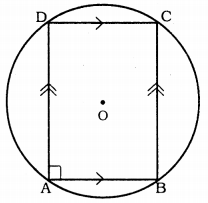Solution:
ABCD is a cyclic parallelogram in the circle with ‘O’ centre.
To Prove: ABCD is a rectangle.
Proof: ABCD is a cyclic parallelogram.
∴ AB || DC and AD || BC
∠A = ∠C (Opposite angles of parallelogram)
But, ∠A + ∠C = 180° (Opposites angles of cyclic quadrilateral)
∠A + ∠A = 180°
2∠A = 180°
∠A = 1802
∴ ∠A = 90°
If each angle of the parallelogram is a right angle, it is a rectangle.
∴ ABCD is a rectangle.

All Chapter KSEEB Solutions For Class 9 Maths

—————————————————————————–

All Subject KSEEB Solutions For Class 9

*************************************************

I think you got complete solutions for this chapter. If You have any queries regarding this chapter, please comment on the below section our subject teacher will answer you. We tried our best to give complete solutions so you got good marks in your exam.

If these solutions have helped you, you can also share kseebsolutionsfor.com to your friends.

Best of Luck!!